# Power BI – Values() function

Values() function is a table function that can be explained as – When a column name is given this function returns a single column table of distinct values or when a table name is given as input it returns a table with the same columns(entire table) with all the duplicates and blank rows if present.

## Values function

The syntax used for the values() function is given below:

VALUES(Tablename or Columnname)

For a better understanding let us consider two tables:

1. sales_table: which contains sales information of products in INDIA by the customers in the year 2019. The dataset for sales_table is here.
2. product_table: which contains the information of the shop_names from which the products are bought in INDIA in the year 2019. The dataset for product_table is here.

Below are the screenshots of the sales_table and product_table used: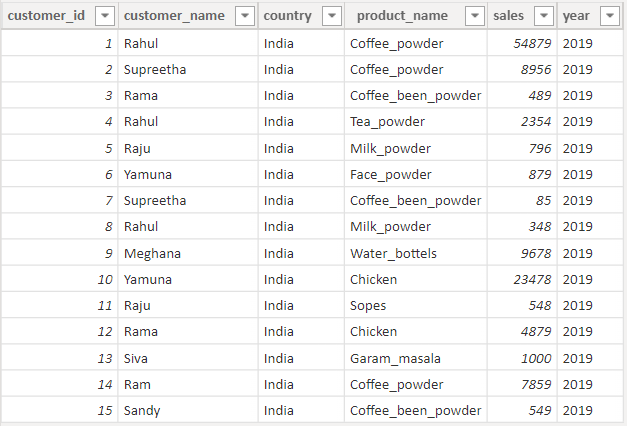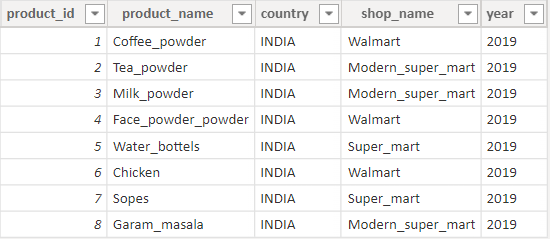Values function will behave differently when tables with relation and without relation. Firstly, Consider sales_table and product_table without relation.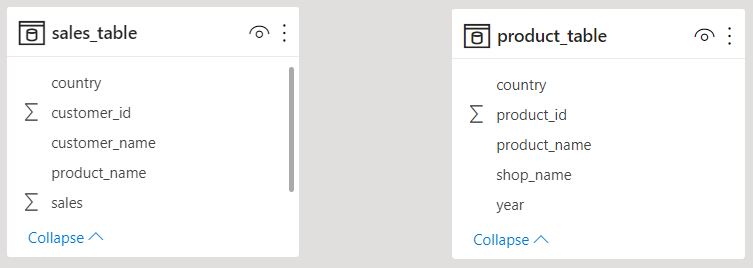Example 1: First let us give the column name as input. Here I am looking for how many distinct values are there in the product_name column in product_table.

DAX code: Values_example1(column_name) = VALUES(product_table[product_name])

If you can observe the output all the distinct values of the product names are listed.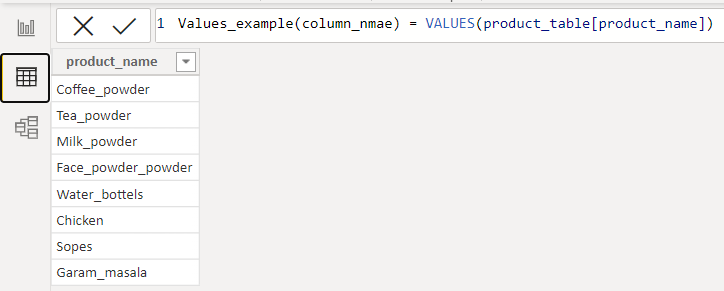Example 2: Next let us give the table name as input,

DAX code: Values_example2(Table_name) = VALUES(product_table)

If you can observe the entire table with the duplicated values is returned as output.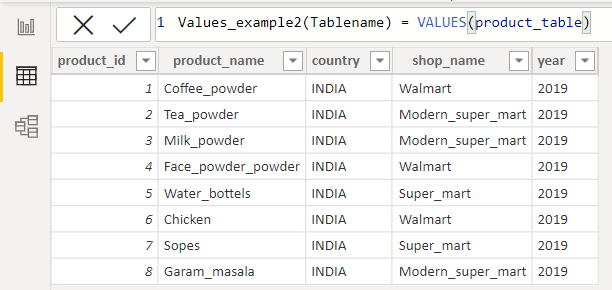Next, we consider the sales_table and product_table with relation. These tables possess one-to-many relations with each other.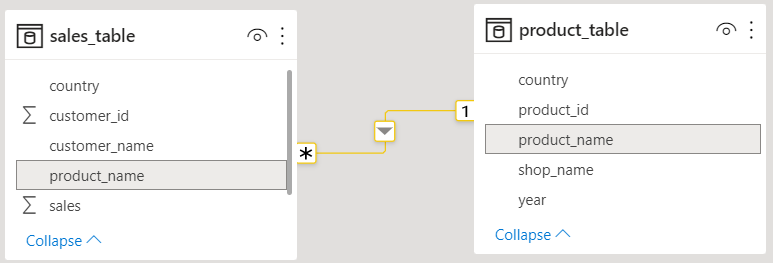Example 1: Same as above. Let us give the column name as input.

DAX code: Values_example1(column_name) = VALUES(product_table[product_name])

As you can observe from the output we have distinct values of the product_name but one empty row is also present in it. Let us check this empty row is present by giving the table name also as input to the values function.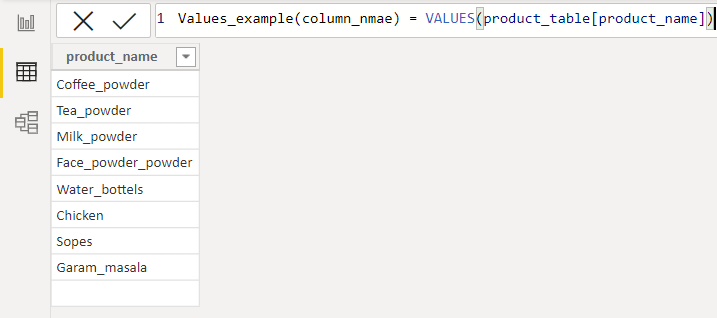Example 2: Let me take the table name as input.

DAX code: Values_example2(Table_name) = VALUES(product_table)

If you can observe from the current output contains an empty row same as the above output.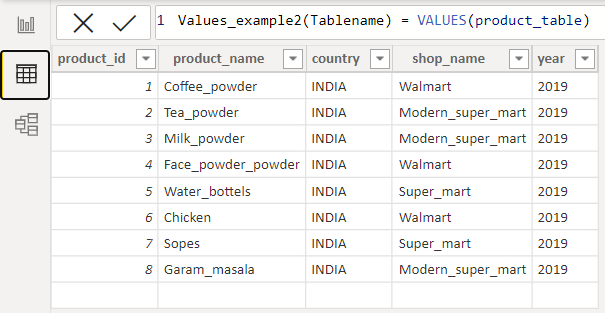This is due to mismatches in the data, if one table is updated with all the values but another related table is not updated properly then this empty row is headed up, in database terminology, it is called a Violation of referential integrity.

Here comes the importance of the values function if you wanted to know the distinct values from the related tables or to know data is updated properly from the related tables then the values function is very useful. When you wanted to become a Data Analyst we should deep dive into the data for proper representation. We need to analyze the data and understand its heart, When I tried to analyze the data and visualize it through the pie chart I got to know that one of the product_name is not updated in the product_table which is present in the sales_table so we are getting an empty row while using this values function.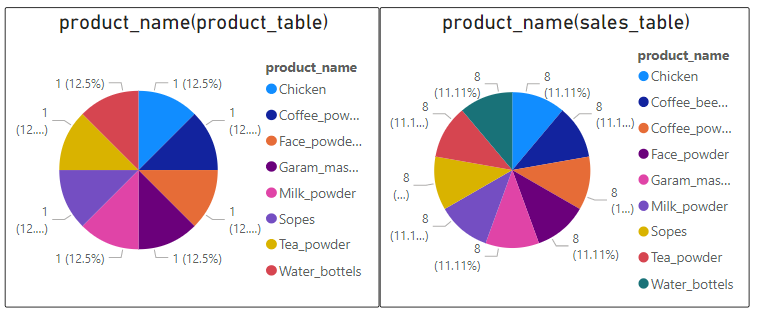Coffee_been_powder is present in the sales_table but it is not updated in the product_table. You can visualize it clearly through the below pie_chart.

### Measures using VALUES Function

Here I have created two measures using the Values function. The first measure is used to calculate the sum of sales for each product in the product_table.

DAX Code: summarize = CALCULATE(SUMX(sales_table,sales_table[sales]),values(product_table[product_name]))

Here we are calculating the sum of sales in the sales_table (using the sumx function) for each product_name in the product_table (using the values function). The output for the above DAX code in matrix format looks like the below :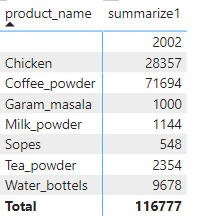Next, the measure is to check the count of products in the sales_table. Here values function is used to collect distinct product_names in the sales_table and countrows are used to calculate the frequency of each product_name.

DAX Code: Number of products in sales table = COUNTROWS(VALUES(sales_table[product_name]))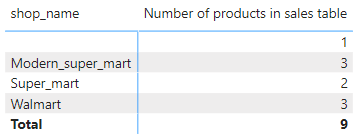If you can observe the above matrix output, the frequency of the product_name according to the shop_name is displayed. If you observe both the outputs have an empty row this is due to a “Violation of referential integrity”.

Whether you're preparing for your first job interview or aiming to upskill in this ever-evolving tech landscape, GeeksforGeeks Courses are your key to success. We provide top-quality content at affordable prices, all geared towards accelerating your growth in a time-bound manner. Join the millions we've already empowered, and we're here to do the same for you. Don't miss out - check it out now!

Previous
Next